# Errata for Butcher and Cotter's The Elements of Nonlinear Optics (Cambridge University Press, 1990)

Errata updated as of March 16, 2003. Additions/corrections welcome!

Below follows a list of errors and misprints found in P. N. Butcher and D. Cotter's The Elements of Nonlinear Optics (Cambridge University Press, Cambridge, 1991), ISBN 0-521-42424-0. Being a summary of the notes I have made in my personal copy of the book since June 1996, this list should by no means be considered as any kind of »official» list of errors, but rather as an attempt to collect the (rather few) misprints in the text. In the list, not only typographical misprints, but also some inconsequent notations—which by no means alter the described theory—are included.

For the lecture notes which resulted from the course that I in 2003 based on Butcher and Cotter's book, please see the separate page where I have collected all lectures.

errata.pdf [107 kB] The errata in PDF format.

errata.djvu [28 kB] The errata in DejaVu format.

errata.ps [178 kB] The errata in PostScript.

errata.tex [12 kB] The errata in plain TeX code. [ download | view source ]

Plug-in viewers of the PDF and DejaVu formats can be downloaded for free from the sites of Adobe and LizardTech, respectively. In order to display PostScript files on screen, you need a viewer like GhostView, which can be downloaded for free from the web site of the University of Wisconsin.

Page 15, lines 14 and 16. In order not to introduce any ambiguity of the multiple arguments of the symmetric and antisymmetric parts, the arguments (t12) should be explicitly written in the left-hand sides of the equations.

Page 15, line 18. " . . .  dummy variables ατ1 and βτ2.'' should be replaced by " . . .  dummy variables (ατ1) and (βτ2).'', following the notation as used later in, for example, §2.3.2 and §4.3.1.

Page 49, line 31. H1(t) should be replaced by HI(t). ("I" for India, not "1".)

Page 54, lines 3, 4, and 6. In Eq. (3.80), the upper limit of integration t1 should be replaced by τ1.

Page 54, lines 8 and 24. In Eqs. (3.81) and (3.82), the upper limits of integration t1 and tn-1 should be replaced by τ1 and τn-1, respectively.

Page 60, line 24. "To achieve this end we . . . " should be replaced by "To achieve this we . . . "

Page 66, line 11. In the right hand side of Eq. (4.49), one should in order not to cause confusion with the Einstein convention of summation over repeated indices explicitly state that no summation is implied, and hence the equation should be written as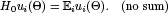using the common notation as used in tensor calculus.

Page 67, line 6. " . . . express the the unperturbed . . . " should be replaced by " . . . express the unperturbed . . . "

Page 72, line 15. "(α,ω1)" should be replaced by "(α,ω1)". (Raise closing parenthesis.)

Page 72, last line. " . . .  of this type, one of which is an identity." should be replaced by " . . .  of this type, of which one is an identity."

Page 86, line 4. In Eq. (4.103), " · · ·  ftn · en>" should be replaced by " · · ·  ftn) · en>".

Page 93, lines 12–13. Strictly speaking, the real part of the susceptibility χ(1)(-ωσ;ω) is not proportional to the refractive index n(ω), but rather to n2(ω)-1.

Page 97, lines 15 and 20. Strictly speaking, Ωfg is the transition angular frequency, and does not have the physical dimension of energy; therefore replace "Ωfg" in lines 15 and 20 by "(h/2π)Ωfg", where h is Planck's constant.

Page 106, line 9. In the first term of Eq. (4.128), the summation should be performed over index j rather than index i, i.e. replace "Σipi" by "Σjpj".

Page 132, line 8. In Eq. (5.30),should be replaced byPage 132, line 25.should be replaced by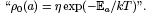(Blackboard strike-through "E" to denote an energy, not the electric field.)

Page 136, line 19. In Eq. (5.43), "χua(1)(-ω,ω)" should be replaced by "χua(1)(-ω,ω)". (Raise "(1)".)

Page 159, line 13. In the left-hand side of Eq. (6.33), the Hamiltonian H0 describing the system is a quantum-mechanical operator, while in the right-hand side, the matrix representation of the corresponding elements <m|H0|n> = δmnEn in the energy representation appears. In order to overcome this inconsistency, Eq. (6.33) should (in analogy with, for example, Eq. (6.31) for the matrix elements of the density operator) be replaced by eitherorPage 160, line 2. In the left-hand side of Eq. (6.34), the Hamiltonian HI(t) is a quantum-mechanical operator, while in the right-hand side, the matrix representation of its scalar elements <a|HI(t)|b> appears. (The same inconsistency appear in Eq. (6.33).) In order to overcome this inconsistency, Eq. (6.34) should (in analogy with, for example, Eq. (6.31) for the matrix elements of the density operator) be replaced by either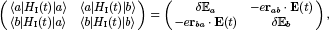orPage 164, line 15. In Eq. (6.49),should be replaced bySee M. D. Levenson and S. S. Kano, Introduction to Nonlinear Laser Spectroscopy (Academic Press, New York, 1988), p. 33, Eqs. (2.3.1)–(2.3.2).

Page 203, lines 32—33. \erritem "Fig. 4.3" should be replaced by "Fig. 4.4(a)".

Page 215, line 11. In Eq. (7.14),should be replaced byPage 220, section 7.2.1. In the example of second harmonic generation, the wave equation (7.26) is given without any explanation of which point symmetry classes of media it applies to, and hence it is from the text virtually impossible to relate the effective nonlinear parameters to the elements of χ(2)\mu\alpha\beta(-ωσ;ω,ω).

Page 234, line 31. In Eq. (7.45),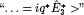should be replaced by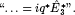Page 240, line 6. In the first line of Eq. (7.55), there is an ambiguity of the denominator as well as an erroneous dispersion term, and the equationshould be replaced by(The other lines of Eq. (7.55) are correct.)

Page 241, line 30. The fundamental bright soliton solution to the nonlinear Schrödinger equation should yieldPage 251, line 6. In Eq. (8.5), there is parenthesis mismatch in both right- and lefthand sides, andshould be replaced byPage 252, line 6. In Eq. (8.7), nphs(q)) should be replaced by nphs(q)], in order to follow the functional style of notation as used in, for example, Eq. (8.5).

Page 253, line 20. In Eq. (8.11), insert a "]" after En(k).

Page 298, Table A3.2. " . . . no centre of symmetry . . . " should be replaced by " . . . no centre of inversion . . . "

Page 317, lines 1, 9, and 24 In Appendix 9, there is an inconsistency in the notation for the polarisation density and the electric dipole operator, as compared to the one used in Chapters 3 and 4. While PD, PQ, and MD in Eq. (A9.1) (and in line 9 on the same page) denote the all-classical electric dipolar, electric quadrupolar and magnetic dipolar polarization densities of the medium, they in Eqs. (A9.6) and (A9.7) clearly denote quantum-mechanical operators. In order to overcome this inconsistency in notation, which in addition gives a wrong answer if properly inserted into the perturbation calculus etc., one should chose either of the conventions. By choosing PD, PQ, and MD to denote the corresponding quantum-mechanical operators, which seems to be the easiest way of correcting this inconsistency, the following corrections to the text should be made:

[line 1]:should be replaced by[line 9]: Remove "PD" or replace with "<PD>".

[line 24]: Somewhere in Appendix 9, there should be a clarifying statement that the nabla operator appearing in Eq. (A9.7) only is operating on the all-classical, macroscopic electric field of the light, and hence should be regarded as a classical vector when evaluating the quantum-mechanical trace that is involved in the expectation value of, for example, the corrected form of Eq. (A9.1).

Page 318, line 12.should be replaced byPage 318, line 23.should be replaced by the same expression, though with each term divided by the value of the elementary charge, e.

Page 333, line 5. In reference Manley, J. M. and Rowe, H. E. (1956), the page numbers should yield 904—14.

Page 334, line 44. "Terhune, R. W. and Weinburger, D. A. . . . " should be replaced by "Terhune, R. W. and Weinberger, D. A. . . . "